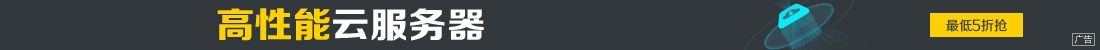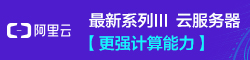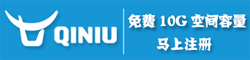# PyTorch中Tensor的拼接与拆分的实现

••1. torch.cat(inputs, dimension=0) → Tensor

```>>> import torch
>>> x = torch.randn(2, 3)
>>> x
tensor([[-0.1997, -0.6900, 0.7039],
[ 0.0268, -1.0140, -2.9764]])
>>> torch.cat((x, x, x), 0) # 在 0 维(纵向)进行拼接
tensor([[-0.1997, -0.6900, 0.7039],
[ 0.0268, -1.0140, -2.9764],
[-0.1997, -0.6900, 0.7039],
[ 0.0268, -1.0140, -2.9764],
[-0.1997, -0.6900, 0.7039],
[ 0.0268, -1.0140, -2.9764]])
>>> torch.cat((x, x, x), 1) # 在 1 维(横向)进行拼接
tensor([[-0.1997, -0.6900, 0.7039, -0.1997, -0.6900, 0.7039, -0.1997, -0.6900,
0.7039],
[ 0.0268, -1.0140, -2.9764, 0.0268, -1.0140, -2.9764, 0.0268, -1.0140,
-2.9764]])
>>> y1 = torch.randn(5, 3, 6)
>>> y2 = torch.randn(5, 3, 6)
>>> torch.cat([y1, y2], 2).size()
torch.Size([5, 3, 12])
>>> torch.cat([y1, y2], 1).size()
torch.Size([5, 6, 6])```

• torch.stack(sequence, dim=0)

```>>> x1 = torch.randn(2, 3)
>>> x2 = torch.randn(2, 3)
>>> torch.stack((x1, x2), 0).size() # 在 0 维插入一个维度，进行区分拼接
torch.Size([2, 2, 3])
>>> torch.stack((x1, x2), 1).size() # 在 1 维插入一个维度，进行组合拼接
torch.Size([2, 2, 3])
>>> torch.stack((x1, x2), 2).size()
torch.Size([2, 3, 2])
>>> torch.stack((x1, x2), 0)
tensor([[[-0.3499, -0.6124, 1.4332],
[ 0.1516, -1.5439, -0.1758]],

[[-0.4678, -1.1430, -0.5279],
[-0.4917, -0.6504, 2.2512]]])
>>> torch.stack((x1, x2), 1)
tensor([[[-0.3499, -0.6124, 1.4332],
[-0.4678, -1.1430, -0.5279]],

[[ 0.1516, -1.5439, -0.1758],
[-0.4917, -0.6504, 2.2512]]])
>>> torch.stack((x1, x2), 2)
tensor([[[-0.3499, -0.4678],
[-0.6124, -1.1430],
[ 1.4332, -0.5279]],

[[ 0.1516, -0.4917],
[-1.5439, -0.6504],
[-0.1758, 2.2512]]])```

• torch.split(tensor, split_size, dim=0)

```>>> x = torch.randn(3, 10, 6)
>>> a, b, c = x.split(1, 0) # 在 0 维进行间隔维 1 的拆分
>>> a.size(), b.size(), c.size()
(torch.Size([1, 10, 6]), torch.Size([1, 10, 6]), torch.Size([1, 10, 6]))
>>> d, e = x.split(2, 0) # 在 0 维进行间隔维 2 的拆分
>>> d.size(), e.size()
(torch.Size([2, 10, 6]), torch.Size([1, 10, 6]))```

• torch.chunk(tensor, chunks, dim=0)

```>>> l, m, n = x.chunk(3, 0) # 在 0 维上拆分成 3 份
>>> l.size(), m.size(), n.size()
(torch.Size([1, 10, 6]), torch.Size([1, 10, 6]), torch.Size([1, 10, 6]))
>>> u, v = x.chunk(2, 0) # 在 0 维上拆分成 2 份
>>> u.size(), v.size()
(torch.Size([2, 10, 6]), torch.Size([1, 10, 6]))```